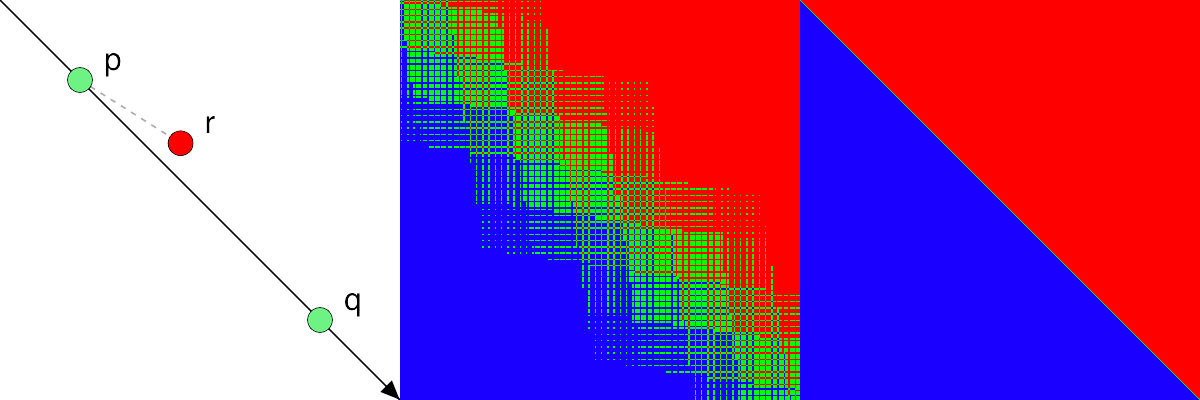Need Package Maintenance

# npm

## robust-predicates2.0.4 • Public • Published

# robust-predicates

Fast robust predicates for computational geometry in JavaScript. Provides reliable 2D and 3D point orientation tests (`orient2d`, `orient3d`, `incircle`, `insphere`) that are not susceptible to floating point errors (without sacrificing performance). A modern port of Jonathan R Shewchuk's C code, an industry standard since 1996.Figure: non-robust vs robust `orient2d` test for points within a tiny range (2-42).

## API

Note: unlike J. Shewchuk's original code, all the functions in this library assume `y` axis is oriented downwards ↓, so the semantics are different.

### `orient2d(ax,ay, bx,by, cx,cy)`

• Returns a positive value if the points `a`, `b`, and `c` occur in counterclockwise order (`c` lies to the left of the directed line defined by points `a` and `b`).
• Returns a negative value if they occur in clockwise order (`c` lies to the right of the directed line `ab`).
• Returns zero if they are collinear.

The result is also an approximation of twice the signed area of the triangle defined by the three points.

### `incircle(ax,ay, bx,by, cx,cy, dx,dy)`

• Returns a positive value if the point `d` lies outside the circle passing through `a`, `b`, and `c`.
• Returns a negative value if it lies inside.
• Returns zero if the four points are cocircular.

The points `a`, `b`, and `c` must be in counterclockwise order, or the sign of the result will be reversed.

### `orient3d(ax,ay,az, bx,by,bz, cx,cy,cz, dx,dy,dz)`

• Returns a positive value if the point `d` lies above the plane passing through `a`, `b`, and `c`, meaning that `a`, `b`, and `c` appear in counterclockwise order when viewed from `d`.
• Returns a negative value if `d` lies below the plane.
• Returns zero if the points are coplanar.

The result is also an approximation of six times the signed volume of the tetrahedron defined by the four points.

### `insphere(ax,ay,az, bx,by,bz, cx,cy,cz, dx,dy,dz, ex,ey,ez)`

• Returns a positive value if the point `e` lies outside the sphere passing through `a`, `b`, `c`, and `d`.
• Returns a negative value if it lies inside.
• Returns zero if the five points are cospherical.

The points `a`, `b`, `c`, and `d` must be ordered so that they have a positive orientation (as defined by `orient3d`), or the sign of the result will be reversed.

### `orient2dfast`, `orient3dfast`, `incirclefast`, `inspherefast`

Simple, approximate, non-robust versions of predicates above. Use when robustness isn't needed.

## Example

```import {orient2d} from 'robust-predicates';

const ccw = orient2d(ax, ay, bx, by, cx, cy) > 0;```

## Install

Install with `npm install robust-predicates` or `yarn add robust-predicates`, or use one of the browser builds:

## Thanks

This project is just a port — all the brilliant, hard work was done by Jonathan Richard Shewchuk.

The port was also inspired by Mikola Lysenko's excellent Robust Arithmetic Notes and related projects like robust-orientation and robust-in-sphere.

Since the original code is in the public domain, this project follows the same choice. See Unlicense.

## Keywords

### Install

`npm i robust-predicates@2.0.4`

### Repository

github.com/mourner/robust-predicates

2.0.4